# Background Estimation (photutils.background)¶

## Introduction¶

To accurately measure the photometry and morphological properties of astronomical sources, one requires an accurate estimate of the background, which can be from both the sky and the detector. Similarly, having an accurate estimate of the background noise is important for determining the significance of source detections and for estimating photometric errors.

Unfortunately, accurate background and background noise estimation is a difficult task. Further, because astronomical images can cover a wide variety of scenes, there is not a single background estimation method that will always be applicable. Photutils provides tools for estimating the background and background noise in your data, but they will likely require some tweaking to optimize the background estimate for your data.

## Scalar Background and Noise Estimation¶

### Simple Statistics¶

If the background level and noise are relatively constant across an image, the simplest way to estimate these values is to derive scalar quantities using simple approximations. Of course, when computing the image statistics one must take into account the astronomical sources present in the images, which add a positive tail to the distribution of pixel intensities. For example, one may consider using the image median as the background level and the image standard deviation as the 1-sigma background noise, but the resulting values are obviously biased by the presence of real sources.

A slightly better method involves using statistics that are robust against the presence of outliers, such as the biweight location for the background level and biweight scale or median absolute deviation (MAD) for the background noise estimation. However, for most astronomical scenes these methods will also be biased by the presence of astronomical sources in the image.

As an example, we load a synthetic image comprised of 100 sources with a Gaussian-distributed background whose mean is 5 and standard deviation is 2:

>>> from photutils.datasets import make_100gaussians_image
>>> data = make_100gaussians_image()


Let’s plot the image:

>>> import matplotlib.pyplot as plt
>>> from astropy.visualization import SqrtStretch
>>> from astropy.visualization.mpl_normalize import ImageNormalize
>>> norm = ImageNormalize(stretch=SqrtStretch())
>>> plt.imshow(data, norm=norm, origin='lower', cmap='Greys_r')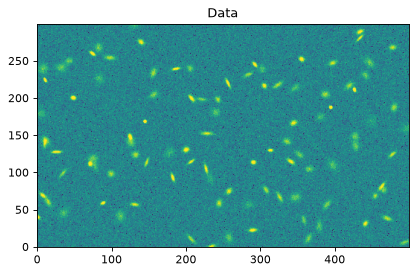The image median and biweight location are both larger than the true background level of 5:

>>> import numpy as np
>>> from astropy.stats import biweight_location
>>> print(np.median(data))
5.225529518399048
>>> print(biweight_location(data))
5.186759755495727


Similarly, using the median absolute deviation to estimate the background noise level gives a value that is larger than the true value of 2:

>>> from astropy.stats import mad_std
2.1443760096598914


### Sigma Clipping Sources¶

The most widely used technique to remove the sources from the image statistics is called sigma clipping. Briefly, pixels that are above or below a specified sigma level from the median are discarded and the statistics are recalculated. The procedure is typically repeated over a number of iterations or until convergence is reached. This method provides a better estimate of the background and background noise levels:

>>> from astropy.stats import sigma_clipped_stats
>>> mean, median, std = sigma_clipped_stats(data, sigma=3.0)
>>> print((mean, median, std))
(5.199138651621793, 5.155587433358291, 2.094275212132969)


An even better procedure is to exclude the sources in the image by masking them. Of course, this technique requires one to identify the sources in the data, which in turn depends on the background and background noise. Therefore, this method for estimating the background and background RMS requires an iterative procedure.

Photutils provides a convenience function, make_source_mask(), for creating source masks. It uses sigma-clipped statistics as the first estimate of the background and noise levels for the source detection. Sources are then identified using image segmentation. Finally, the source masks are dilated to mask more extended regions around the detected sources.

Here we use an aggressive 2-sigma detection threshold to maximize the source detections and dilate using a 11x11 box:

>>> from photutils import make_source_mask
>>> print((mean, median, std))
(5.001013475475569, 5.000584905604376, 1.970887100626572)


Of course, the source detection and masking procedure can be iterated further. Even with one iteration we are within 0.02% of the true background and 1.5% of the true background RMS.

## 2D Background and Noise Estimation¶

If the background or the background noise varies across the image, then you will generally want to generate a 2D image of the background and background RMS (or compute these values locally). This can be accomplished by applying the above techniques to subregions of the image. A common procedure is to use sigma-clipped statistics in each mesh of a grid that covers the input data to create a low-resolution background image. The final background or background RMS image can then be generated by interpolating the low-resolution image.

Photutils provides the Background2D class to estimate the 2D background and background noise in an astronomical image. Background2D requires the size of the box (box_size) in which to estimate the background. Selecting the box size requires some care by the user. The box size should generally be larger than the typical size of sources in the image, but small enough to encapsulate any background variations. For best results, the box size should also be chosen so that the data are covered by an integer number of boxes in both dimensions. If that is not the case, the edge_method keyword determines whether to pad or crop the image such that there is an integer multiple of the box_size in both dimensions.

The background level in each of the meshes is calculated using the function or callable object (e.g. class instance) input via bkg_estimator keyword. Photutils provides a several background classes that can be used:

The default is a SExtractorBackground instance. For this method, the background in each mesh is calculated as (2.5 * median) - (1.5 * mean). However, if (mean - median) / std > 0.3 then the median is used instead (despite what the SExtractor User’s Manual says, this is the method it always uses).

Likewise, the background RMS level in each mesh is calculated using the function or callable object input via the bkgrms_estimator keyword. Photutils provides the following classes for this purpose:

For even more flexibility, users may input a custom function or callable object to the bkg_estimator and/or bkgrms_estimator keywords.

By default, the bkg_estimator and bkgrms_estimator are applied to sigma clipped data. Sigma clipping is defined by inputting a astropy.stats.SigmaClip object to the sigma_clip keyword. The default is to perform sigma clipping with sigma=3 and maxiters=10. Sigma clipping can be turned off by setting sigma_clip=None.

After the background level has been determined in each of the boxes, the low-resolution background image can be median filtered, with a window of size of filter_size, to suppress local under- or overestimations (e.g., due to bright galaxies in a particular box). Likewise, the median filter can be applied only to those boxes where the background level is above a specified threshold (filter_threshold).

The low-resolution background and background RMS images are resized to the original data size using the function or callable object input via the interpolator keyword. Photutils provides two interpolator classes: BkgZoomInterpolator (default), which performs spline interpolation, and BkgIDWInterpolator, which uses inverse-distance weighted (IDW) interpolation.

For this example, we will create a test image by adding a strong background gradient to the image defined above:

>>> ny, nx = data.shape
>>> y, x = np.mgrid[:ny, :nx]
>>> gradient =  x * y / 5000.
>>> data2 = data + gradient
>>> plt.imshow(data2, norm=norm, origin='lower', cmap='Greys_r')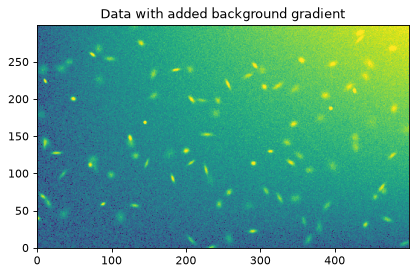We start by creating a Background2D object using a box size of 50x50 and a 3x3 median filter. We will estimate the background level in each mesh as the sigma-clipped median using an instance of MedianBackground.

>>> from astropy.stats import SigmaClip
>>> from photutils import Background2D, MedianBackground
>>> sigma_clip = SigmaClip(sigma=3.)
>>> bkg_estimator = MedianBackground()
>>> bkg = Background2D(data2, (50, 50), filter_size=(3, 3),
...                    sigma_clip=sigma_clip, bkg_estimator=bkg_estimator)


The 2D background and background RMS images are retrieved using the background and background_rms attributes, respectively, on the returned object. The low-resolution versions of these images are stored in the background_mesh and background_rms_mesh attributes, respectively. The global median value of the low-resolution background and background RMS image can be accessed with the background_median and background_rms_median attributes, respectively:

>>> print(bkg.background_median)
10.821997862561792
>>> print(bkg.background_rms_median)
2.298820539683762


Let’s plot the background image:

>>> plt.imshow(bkg.background, origin='lower', cmap='Greys_r')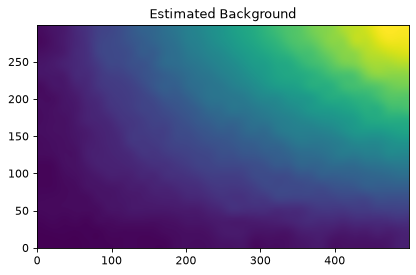and the background-subtracted image:

>>> plt.imshow(data2 - bkg.background, norm=norm, origin='lower',
...            cmap='Greys_r')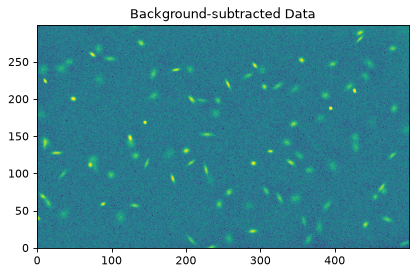Masks can also be input into Background2D. As described above, this can be employed to mask sources in the image prior to estimating the background levels.

Additionally, input masks are often necessary if your data array includes regions without data coverage (e.g., from a rotated image or an image from a mosaic). Otherwise, the data values in the regions without coverage (usually zeros or NaNs) will adversely contribute to the background statistics.

Let’s create such an image and plot it (NOTE: this example requires scipy):

>>> from scipy.ndimage import rotate
>>> data3 = rotate(data2, -45.)
>>> norm = ImageNormalize(stretch=SqrtStretch())
>>> plt.imshow(data3, origin='lower', cmap='Greys_r', norm=norm)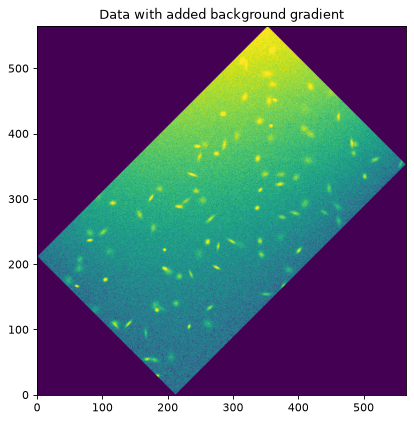Now we create a coverage mask and input it into Background2D to exclude the regions where we have no data. For real data, one can usually create a coverage mask from a weight or noise image. In this example we also use a smaller box size to help capture the strong gradient in the background:

>>> mask = (data3 == 0)


The input masks are never applied to the returned background image because the input mask can represent either a coverage mask or a source mask, or a combination of both. Therefore, we need to manually apply the coverage mask to the returned background image:

>>> back3 = bkg3.background * ~mask
>>> norm = ImageNormalize(stretch=SqrtStretch())
>>> plt.imshow(back3, origin='lower', cmap='Greys_r', norm=norm)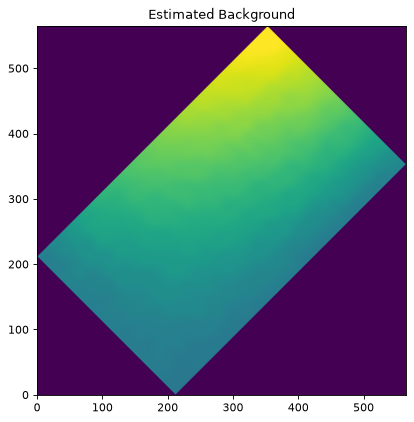Finally, let’s subtract the background from the image and plot it:

>>> norm = ImageNormalize(stretch=SqrtStretch())
>>> plt.imshow(data3 - back3, origin='lower', cmap='Greys_r', norm=norm)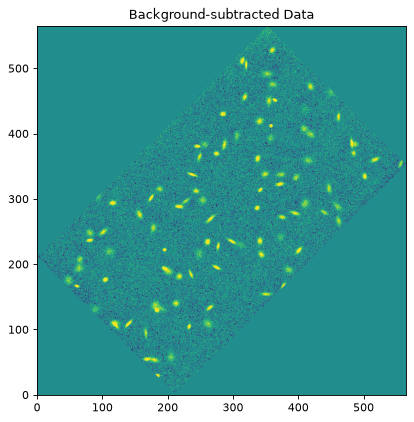If there is any small residual background still present in the image, the background subtraction can be improved by masking the sources and/or through further iterations.

### Plotting Meshes¶

Finally, the meshes that were used in generating the 2D background can be plotted on the original image using the plot_meshes() method:

>>> plt.imshow(data3, origin='lower', cmap='Greys_r', norm=norm)
>>> bkg3.plot_meshes(outlines=True, color='#1f77b4')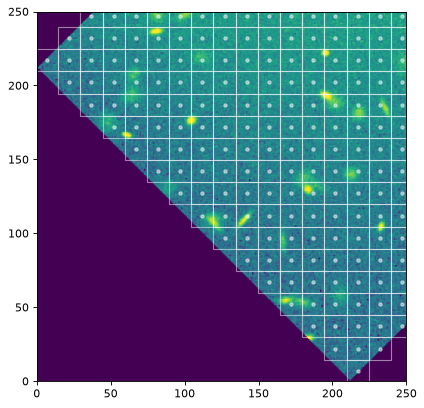The meshes extended beyond the original image on the top and right because Background2D’s default edge_method is 'pad'.

## Reference/API¶

This subpackage contains tools to estimate the background and background RMS in an image.

### Classes¶

 Background2D(data, box_size[, mask, …]) Class to estimate a 2D background and background RMS noise in an image. BackgroundBase([sigma_clip]) Base class for classes that estimate scalar background values. BackgroundRMSBase([sigma_clip]) Base class for classes that estimate scalar background RMS values. Class to calculate the background in an array using the biweight location. Class to calculate the background RMS in an array as the (sigma-clipped) biweight scale. BkgIDWInterpolator([leafsize, n_neighbors, …]) This class generates full-sized background and background RMS images from lower-resolution mesh images using inverse-distance weighting (IDW) interpolation (ShepardIDWInterpolator). BkgZoomInterpolator([order, mode, cval]) This class generates full-sized background and background RMS images from lower-resolution mesh images using the zoom (spline) interpolator. MADStdBackgroundRMS([sigma_clip]) Class to calculate the background RMS in an array as using the median absolute deviation (MAD). MMMBackground(**kwargs) Class to calculate the background in an array using the DAOPHOT MMM algorithm. MeanBackground([sigma_clip]) Class to calculate the background in an array as the (sigma-clipped) mean. MedianBackground([sigma_clip]) Class to calculate the background in an array as the (sigma-clipped) median. ModeEstimatorBackground([median_factor, …]) Class to calculate the background in an array using a mode estimator of the form (median_factor * median) - (mean_factor * mean). SExtractorBackground([sigma_clip]) Class to calculate the background in an array using the SExtractor algorithm. StdBackgroundRMS([sigma_clip]) Class to calculate the background RMS in an array as the (sigma-clipped) standard deviation.